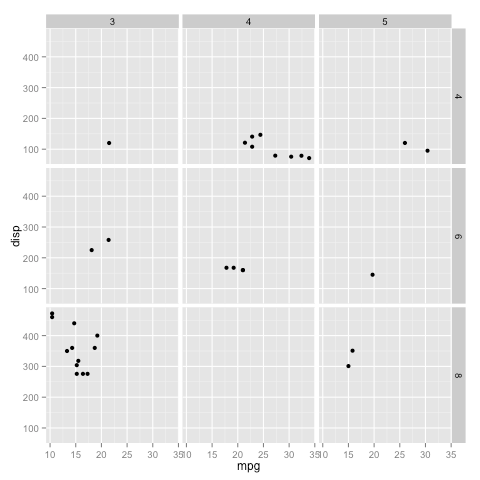[This article was first published on DataScience+, and kindly contributed to R-bloggers]. (You can report issue about the content on this page here)
Want to share your content on R-bloggers? click here if you have a blog, or here if you don't.

In this article, I will show you how to use the `ggplot2` plotting library in R. It was written by Hadley Wickham. If you don’t have already have it, install it and load it up:

```install.packages('ggplot2')
library(ggplot2)
```

## qplot

`qplot` is the quickest way to get off the ground running. For this demonstration, we will use the `mtcars` dataset from the `datasets` package.

```library(datasets)
qplot(mpg, disp, data = mtcars)```

We can also color the datapoints based on the number of cylinders that each car has as follows:

```mtcars\$cyl <- as.factor(mtcars\$cyl)
qplot(mpg, disp, data = mtcars, color = cyl)```

You can also plot a histogram:

```qplot(mtcars\$mpg, fill = mtcars\$cyl, binwidth = 2)
```

Another thing you may notice is that instead of specifying `data = mtcars`, I just used `mtcars\$mpg` and `mtcars\$cyl` here. Both are acceptable ways, and you are free to use whichever you prefer.

You can also split the plot using facets.

```qplot(mpg, disp, data = mtcars, facets = cyl ~ .)
```

You can also split along both the x axes and y axes as follows:

```mtcars\$gear <- as.factor(mtcars\$gear)
qplot(mpg, disp, data - mtcars, facets = cyl ~ gear)
```## ggplot

While `qplot` is a great way to get off the ground running, it does not provide the same level of customization as `ggplot`. All the above plots can be reproduced using `ggplot` as follows:

```ggplot(mtcars, aes(mpg, disp)) + geom_point()
ggplot(mtcars, aes(mpg, disp)) + geom_point(aes(color = cyl)
ggplot(mtcars, aes(mpg)) + geom_bar(aes(fill = cyl), binwidth = 2)
ggplot(mtcars, aes(mpg, disp)) + geom_point() + facet_grid(cyl ~ .)
ggplot(mtcars, aes(mpg, disp)) + geom_point() + facet_grid(cyl ~ gear)
```

### Customization

There are a variety of options available for customization. I will describe a few here.

For example, for the points, we can specify size, color and alpha. Alpha determines how opaque each point is, with 0 being the lowest, and 1 being the highest value it can take.

We can specify the labels for the x axis and y axis using `xlab` and `ylab` respectively, and the title using `ggtitle`.

There are a variety of options for modifying the legend title, text, colors, order, position, etc.

You can also select a theme for the plot. Use `?ggtheme` to see all the options that are available.

Here is an example:

```ggplot(mtcars, aes(mpg, disp)) +
geom_point(aes(color = carb), size = 2.5, alpha = 0.8) +
facet_grid(cyl ~ gear) +
xlab('Miles per US gallon') +
ylab('Displacement in cubic inches') +
ggtitle('Fuel consumption vs displacement') +
theme(legend.background = element_rect(colour = 'orange', fill = 'purple', size = 1.2, linetype = 'dotted'), legend.key = element_rect(fill = 'pink'), legend.position = 'top')
```

Please note the spelling of “colour” in the legend settings. “Color” will not work.

The above plot is only for demonstration purposes, and it shows some of the many customization options available in the `ggplot2` library. For more options, please refer to the ggplot2 documentation.

If you have any questions, please feel free to leave a comment or reach out to me on Twitter.